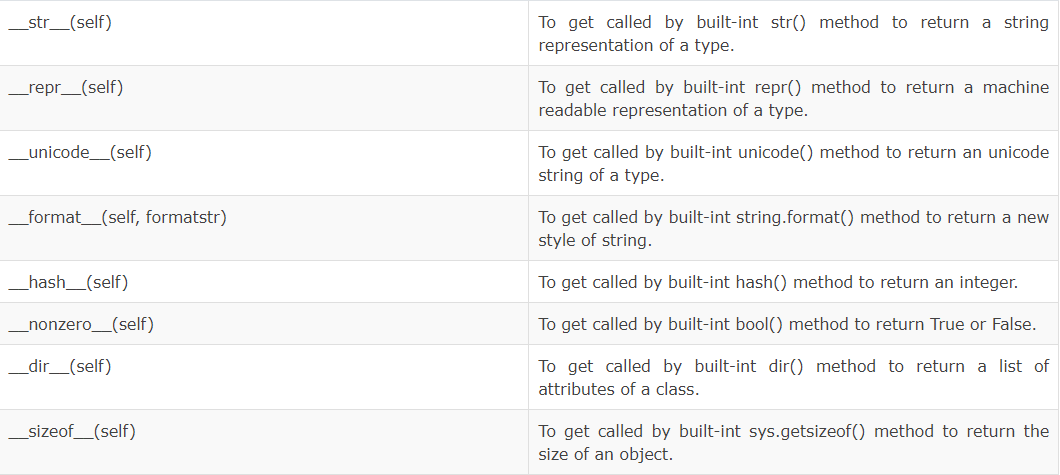Consider the following code,

# defining a student class
class Student:
def __init__(self,maths,phy):
self.maths = maths
self.phy = phy

# the special method in python
def __str__(self):
return f"The math marks is {self.maths}, and the physics marks is {self.phy}"

# declaring two students along with the marks
monty = Student(75,80)
parker = Student(32,78)

print(monty + parker)

---------------------------------------------------------------------------
TypeError                                 Traceback (most recent call last)
<ipython-input-5-7af7a86df8ea> in <module>
10 parker = Student(32,78)
11
---> 12 print(monty + parker)

TypeError: unsupported operand type(s) for +: 'Student' and 'Student'


It created a TypeError !!

This error is generated, because we have used an inbuilt operator + that’s not designed to add two objects, just like we have seen in the special function segment (the print() function). Python has special functions to carry out operator overloading and modify the behavior of the inbuilt operators.

To overload the + operator, we will need to implement __add__() function in the class.

# defining a student class
class Student:
def __init__(self,maths,phy):
self.maths = maths
self.phy = phy

# the special method in python
def __str__(self):
return f"The math marks is {self.maths}, and the physics marks is {self.phy}"

maths = self.maths + other.maths
phy = self.phy + other.phy
return Student(maths,phy)

# declaring two students along with the marks
monty = Student(75,80)
parker = Student(32,78)

# printing the sum of the marks
print(monty + parker)


What actually happens is that, when you use monty + parker, Python calls monty.__add__(parker) which in turn is Student.__add__(monty,parker). After this, the addition operation is carried out the way we specified.

Similarly, we can overload other operators as well. The special function that we need to implement is tabulated below.Q - Design a program to take two fruit objects and compare their price using operator overloading.
Q - Design a program to take 10 student objects and and rank them according to their percentage using operator overloading.